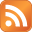# Testing multicast is working with omping

Published on August 10th 2016 - Listed in Linux Network

Needed to see if multicast connections between two hosts were working. omping is designed to do this for you:

"The omping is program which uses User Datagram Protocol to determine if computer is able to send and/or receive IP unicast and multicast or Broadcast packets from the network."

In my case I needed to see if two CentOS 7 hosts are able to communicate with each other with multicast. On each host the program omping was installed:

yum install omping

And then omping is launched on both hosts, where -m 238.0.0.1 represents the multicast broadcast address (check your multicast settings):

omping -m 238.0.0.1 host1 host2

The output on both hosts should show the omping replies from the other host:

[root@host1 ~]# omping -m 238.0.0.1 host1 host2
host2 : waiting for response msg
host2 : joined (S,G) = (*, 232.43.211.234), pinging
host2 :   unicast, seq=1, size=69 bytes, dist=0, time=0.122ms
host2 :   unicast, seq=2, size=69 bytes, dist=0, time=0.258ms
host2 : multicast, seq=2, size=69 bytes, dist=0, time=0.371ms
host2 :   unicast, seq=3, size=69 bytes, dist=0, time=0.328ms
host2 : multicast, seq=3, size=69 bytes, dist=0, time=0.434ms
host2 :   unicast, seq=4, size=69 bytes, dist=0, time=0.249ms
host2 : multicast, seq=4, size=69 bytes, dist=0, time=0.374ms
host2 :   unicast, seq=5, size=69 bytes, dist=0, time=0.270ms
host2 : multicast, seq=5, size=69 bytes, dist=0, time=0.379ms
host2 :   unicast, seq=6, size=69 bytes, dist=0, time=0.240ms
host2 : multicast, seq=6, size=69 bytes, dist=0, time=0.348ms
host2 :   unicast, seq=7, size=69 bytes, dist=0, time=0.272ms

[root@host2 ~]# omping -m 238.0.0.1 host1 host2
host1 : waiting for response msg
host1 : waiting for response msg
host1 : joined (S,G) = (*, 232.43.211.234), pinging
host1 :   unicast, seq=1, size=69 bytes, dist=0, time=0.116ms
host1 : multicast, seq=1, size=69 bytes, dist=0, time=0.235ms
host1 :   unicast, seq=2, size=69 bytes, dist=0, time=0.219ms
host1 : multicast, seq=2, size=69 bytes, dist=0, time=0.329ms
host1 :   unicast, seq=3, size=69 bytes, dist=0, time=0.700ms
host1 : multicast, seq=3, size=69 bytes, dist=0, time=0.800ms
host1 :   unicast, seq=4, size=69 bytes, dist=0, time=0.249ms
host1 : multicast, seq=4, size=69 bytes, dist=0, time=0.360ms
host1 :   unicast, seq=5, size=69 bytes, dist=0, time=0.232ms
host1 : multicast, seq=5, size=69 bytes, dist=0, time=0.323ms
host1 :   unicast, seq=6, size=69 bytes, dist=0, time=0.291ms
host1 : multicast, seq=6, size=69 bytes, dist=0, time=0.377ms
host1 :   unicast, seq=7, size=69 bytes, dist=0, time=0.291ms
host1 : multicast, seq=7, size=69 bytes, dist=0, time=0.382ms

Multicast works between these two hosts running in the same data center.

Now I added a third host, running outside of the data center. Which means the traffic needs to go through a couple of routers and switches. Will multicast still work?

[root@host1 ~]# omping -m 238.0.0.1 host1 host2 host3
host2 : waiting for response msg
host3 : waiting for response msg
host2 : waiting for response msg
host3 : waiting for response msg
host2 : joined (S,G) = (*, 232.43.211.234), pinging
host2 :   unicast, seq=1, size=69 bytes, dist=0, time=0.158ms
host2 : multicast, seq=1, size=69 bytes, dist=0, time=0.283ms
host3 : waiting for response msg
host2 :   unicast, seq=2, size=69 bytes, dist=0, time=0.199ms
host2 : multicast, seq=2, size=69 bytes, dist=0, time=0.323ms
host3 : joined (S,G) = (*, 232.43.211.234), pinging
host3 :   unicast, seq=1, size=69 bytes, dist=6, time=4.050ms
host2 :   unicast, seq=3, size=69 bytes, dist=0, time=0.267ms
host2 : multicast, seq=3, size=69 bytes, dist=0, time=0.382ms
host3 :   unicast, seq=2, size=69 bytes, dist=6, time=3.990ms
host2 :   unicast, seq=4, size=69 bytes, dist=0, time=0.299ms
host2 : multicast, seq=4, size=69 bytes, dist=0, time=0.308ms
host3 :   unicast, seq=3, size=69 bytes, dist=6, time=4.110ms

[root@host2 ~]# omping -m 238.0.0.1 host1 host2 host3
host1 : waiting for response msg
host3 : waiting for response msg
host1 : joined (S,G) = (*, 232.43.211.234), pinging
host1 :   unicast, seq=1, size=69 bytes, dist=0, time=0.146ms
host1 :   unicast, seq=2, size=69 bytes, dist=0, time=0.229ms
host1 : multicast, seq=2, size=69 bytes, dist=0, time=0.338ms
host3 : waiting for response msg
host1 :   unicast, seq=3, size=69 bytes, dist=0, time=0.314ms
host1 : multicast, seq=3, size=69 bytes, dist=0, time=0.436ms
host3 : joined (S,G) = (*, 232.43.211.234), pinging
host3 :   unicast, seq=1, size=69 bytes, dist=6, time=4.108ms
host1 :   unicast, seq=4, size=69 bytes, dist=0, time=0.208ms
host1 : multicast, seq=4, size=69 bytes, dist=0, time=0.316ms
host3 :   unicast, seq=2, size=69 bytes, dist=6, time=3.981ms
host1 :   unicast, seq=5, size=69 bytes, dist=0, time=0.257ms

claudio@host3 ~ omping -m 238.0.0.1 host1 host2 host3
host1 : waiting for response msg
host2 : waiting for response msg
host1 : joined (S,G) = (*, 232.43.211.234), pinging
host2 : joined (S,G) = (*, 232.43.211.234), pinging
host2 :   unicast, seq=1, size=69 bytes, dist=6, time=3.948ms
host1 :   unicast, seq=1, size=69 bytes, dist=6, time=3.985ms
host1 :   unicast, seq=2, size=69 bytes, dist=6, time=4.076ms
host2 :   unicast, seq=2, size=69 bytes, dist=6, time=4.072ms
host2 :   unicast, seq=3, size=69 bytes, dist=6, time=4.072ms
host1 :   unicast, seq=3, size=69 bytes, dist=6, time=4.112ms
host1 :   unicast, seq=4, size=69 bytes, dist=6, time=4.045ms
host2 :   unicast, seq=4, size=69 bytes, dist=6, time=4.056ms

While multicast between host1 and host2 still works (as one can see in the replies), all connections to host3 only work using unicast. In this case multicast connectivity to host3 does not work.

Update January 18th 2018: I was missing the multicast broadcast address in the command to correctly verify multicast. I added it in the command (-m address).